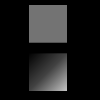# Py5Shape.normal()#

Sets the current normal vector for a Py5Shape object’s vertices.

## Examples#def setup():
py5.size(100, 100, py5.P3D)
py5.background(0)
py5.directional_light(255, 255, 255, -1, -1, -1)

s1 = py5.create_shape()
s1.begin_shape()
s1.fill(200, 200, 200)
s1.vertex(-20, -20, -25)
s1.vertex(20, -20, -25)
s1.vertex(20, 20, -25)
s1.vertex(-20, 20, -25)
s1.end_shape(py5.CLOSE)

s2 = py5.create_shape()
s2.begin_shape()
s2.fill(200, 200, 200)
s2.normal(-20, -20, 20)
s2.vertex(-20, -20, -25)
s2.normal(20, -20, 20)
s2.vertex(20, -20, -25)
s2.normal(20, 20, 20)
s2.vertex(20, 20, -25)
s2.normal(-20, 20, 20)
s2.vertex(-20, 20, -25)
s2.end_shape(py5.CLOSE)

py5.shape(s1, 40, 10)
py5.shape(s2, 40, 60)


## Description#

Sets the current normal vector for a Py5Shape object’s vertices. Used for drawing three dimensional shapes and surfaces, normal() specifies a vector perpendicular to a shape’s surface which, in turn, determines how lighting affects it. Py5 attempts to automatically assign normals to shapes, but since that’s imperfect, this is a better option when you want more control.

This method can only be used within a Py5Shape.begin_shape() and Py5Shape.end_shape() pair. The normal setting will be applied to vertices added after the call to this method.

Underlying Processing method: PShape.normal

## Signatures#

normal(
nx: float,  # x direction
ny: float,  # y direction
nz: float,  # z direction
/,
) -> None


Updated on March 06, 2023 02:49:26am UTC